Theory and Modern Applications

# Basins of attraction of certain rational anti-competitive system of difference equations in the plane

## Abstract

We investigate the global asymptotic behavior of solutions of the following anti-competitive system of rational difference equations:

${x}_{n+1}=\frac{{\gamma }_{1}{y}_{n}}{{A}_{1}+{x}_{n}},\phantom{\rule{2em}{0ex}}{y}_{n+1}=\frac{{\beta }_{2}{x}_{n}}{{A}_{2}+{B}_{2}{x}_{n}+{y}_{n}},\phantom{\rule{1em}{0ex}}n=0,1,\dots ,$

where the parameters ${\gamma }_{1}$, ${\beta }_{2}$, ${A}_{1}$, ${A}_{2}$ and ${B}_{2}$ are positive numbers and the initial conditions $\left({x}_{0},{y}_{0}\right)$ are arbitrary nonnegative numbers. We find the basins of attraction of all attractors of this system, which are the equilibrium points and period-two solutions.

MSC:39A10, 39A11.

## 1 Introduction

A first-order system of difference equations

$\left\{\begin{array}{c}{x}_{n+1}=f\left({x}_{n},{y}_{n}\right),\\ {y}_{n+1}=g\left({x}_{n},{y}_{n}\right),\end{array}\phantom{\rule{1em}{0ex}}n=0,1,\dots ,\left({x}_{0},{y}_{0}\right)\in \mathcal{R},$
(1)

where $\mathcal{R}\subset {\mathbb{R}}^{2}$, $\left(f,g\right):\mathcal{R}\to \mathcal{R},f,g$ are continuous functions is competitive if $f\left(x,y\right)$ is non-decreasing in x and non-increasing in y, and $g\left(x,y\right)$ is non-increasing in x and non-decreasing in y.

System (1) where the functions f and g have a monotonic character opposite of the monotonic character in competitive system will be called anti-competitive.

We consider the following anti-competitive system of difference equations:

${x}_{n+1}=\frac{{\gamma }_{1}{y}_{n}}{{A}_{1}+{x}_{n}},\phantom{\rule{2em}{0ex}}{y}_{n+1}=\frac{{\beta }_{2}{x}_{n}}{{A}_{2}+{B}_{2}{x}_{n}+{y}_{n}},\phantom{\rule{1em}{0ex}}n=0,1,\dots ,$
(2)

where the parameters ${A}_{1}$, ${\gamma }_{1}$, ${A}_{2}$, ${B}_{2}$ and ${\beta }_{2}$ are positive numbers and the initial conditions $\left({x}_{0},{y}_{0}\right)$ are arbitrary nonnegative numbers. In the classification of all linear fractional systems in , System (2) was mentioned as System (16, 39).

Competitive and cooperative systems of the form (1) were studied by many authors such as Clark and Kulenović , Clark, Kulenović and Selgrade , Hirsch and Smith , Kulenović and Ladas , Kulenović and Merino , Kulenović and Nurkanović [7, 8], Garić-Demirović, Kulenović and Nurkanović [9, 10], Smith [11, 12] and others.

The study of anti-competitive systems started in  and has advanced since then (see [14, 15]). The principal tool of the study of anti-competitive systems is the fact that the second iterate of the map associated with an anti-competitive system is a competitive map, and so the elaborate theory for such maps developed recently in [4, 16, 17] can be applied.

The main result on the global behavior of System (2) is the following theorem.

Theorem 1 (a) If ${\beta }_{2}{\gamma }_{1}\le {A}_{1}{A}_{2}$, then ${E}_{0}=\left(0,0\right)$ is a unique equilibrium, and the basin of attraction of this equilibrium is $\mathcal{B}\left({E}_{0}\right)=\left\{\left(x,y\right):x\ge 0,y\ge 0\right\}$ (see Figure 1(a)).

(b) If ${\beta }_{2}{\gamma }_{1}-{A}_{1}{A}_{2}>-{B}_{2}\left[{A}_{1}^{2}+{\gamma }_{1}\left({A}_{2}-{A}_{1}{B}_{2}\right)\right]$ and ${\beta }_{2}{\gamma }_{1}-{A}_{1}{A}_{2}>0$, then there exist two equilibrium points: ${E}_{0}$ which is a repeller and ${E}_{+}$ which is an interior saddle point, and minimal period-two solutions ${A}_{0}=\left(0,\frac{{\beta }_{2}{\gamma }_{1}-{A}_{1}{A}_{2}}{{\gamma }_{1}{B}_{2}}\right)$ and ${B}_{0}=\left(\frac{{\beta }_{2}{\gamma }_{1}-{A}_{1}{A}_{2}}{{A}_{1}{B}_{2}},0\right)$ which are locally asymptotically stable. There exists a set $\mathcal{C}\subset \mathcal{R}=\left[0,\mathrm{\infty }\right)×\left[0,\mathrm{\infty }\right)$ such that ${E}_{0}\in \mathcal{C}$, and ${\mathcal{W}}^{s}\left({E}_{+}\right)=\mathcal{C}\setminus {E}_{0}$ is an invariant subset of the basin of attraction of ${E}_{+}$. The set $\mathcal{C}$ is a graph of a strictly increasing continuous function of the first variable on an interval and separates $\mathcal{R}$ into two connected and invariant components, namely

${\mathcal{W}}_{-}:=\left\{\mathbf{x}\in \mathcal{R}\mathrm{\setminus }\mathcal{C}:\mathrm{\exists }{\mathbf{x}}^{\mathrm{\prime }}\in \mathcal{C}\phantom{\rule{0.25em}{0ex}}\mathit{\text{with}}\phantom{\rule{0.25em}{0ex}}\mathbf{x}{⪯}_{se}{\mathbf{x}}^{\mathrm{\prime }}\right\},\phantom{\rule{2em}{0ex}}{\mathcal{W}}_{+}:=\left\{\mathbf{x}\in \mathcal{R}\mathrm{\setminus }\mathcal{C}:\mathrm{\exists }{\mathbf{x}}^{\mathrm{\prime }}\in \mathcal{C}\phantom{\rule{0.25em}{0ex}}\mathit{\text{with}}\phantom{\rule{0.25em}{0ex}}{\mathbf{x}}^{\mathrm{\prime }}{⪯}_{se}\mathbf{x}\right\},$

which satisfy (see Figure 1(b)):

(i) If $\left({x}_{0},{y}_{0}\right)\in {\mathcal{W}}_{+}$, then

$\underset{n\to \mathrm{\infty }}{lim}\left({x}_{2n},{y}_{2n}\right)=\left(\frac{{\beta }_{2}{\gamma }_{1}-{A}_{1}{A}_{2}}{{A}_{1}{B}_{2}},0\right)={B}_{0}$

and

$\underset{n\to \mathrm{\infty }}{lim}\left({x}_{2n+1},{y}_{2n+1}\right)=\left(0,\frac{{\beta }_{2}{\gamma }_{1}-{A}_{1}{A}_{2}}{{\gamma }_{1}{B}_{2}}\right)={A}_{0}.$

(ii) If $\left({x}_{0},{y}_{0}\right)\in {\mathcal{W}}_{-}$, then

$\underset{n\to \mathrm{\infty }}{lim}\left({x}_{2n},{y}_{2n}\right)=\left(0,\frac{{\beta }_{2}{\gamma }_{1}-{A}_{1}{A}_{2}}{{\gamma }_{1}{B}_{2}}\right)={A}_{0}$

and

$\underset{n\to \mathrm{\infty }}{lim}\left({x}_{2n+1},{y}_{2n+1}\right)=\left(\frac{{\beta }_{2}{\gamma }_{1}-{A}_{1}{A}_{2}}{{A}_{1}{B}_{2}},0\right)={B}_{0}.$

(c) If $0<{\beta }_{2}{\gamma }_{1}-{A}_{1}{A}_{2}=-{B}_{2}\left[{A}_{1}^{2}+{\gamma }_{1}\left({A}_{2}-{A}_{1}{B}_{2}\right)\right]$, then (see Figure 1(c))

(i) There exist two equilibrium points: ${E}_{0}$ which is a repeller and ${E}_{+}\in int\left(\mathcal{R}\right)$ which is a non-hyperbolic, and an infinite number of minimal period-two solutions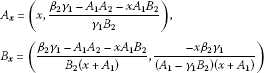for $x\in \left[0,\frac{{\beta }_{2}{\gamma }_{1}-{A}_{1}{A}_{2}}{{A}_{1}{B}_{2}}\right]$, that belong to the segment of the line (15) in the first quadrant.

(ii) All minimal period-two solutions and the equilibrium ${E}_{+}$ are stable but not asymptotically stable.

(iii) There exists a family of strictly increasing curves ${\mathcal{C}}_{+}$, ${\mathcal{C}}_{{A}_{x}}$, ${\mathcal{C}}_{{B}_{x}}$ for $x\in \left(0,\frac{{\beta }_{2}{\gamma }_{1}-{A}_{1}{A}_{2}}{{A}_{1}{B}_{2}}\right)$ and

${\mathcal{C}}_{{A}_{0}}=\left\{\left(x,y\right):x=0,y>0\right\},\phantom{\rule{2em}{0ex}}{\mathcal{C}}_{{B}_{0}}=\left\{\left(x,y\right):x>0,y=0\right\}$

that emanate from ${E}_{0}$ and ${A}_{x}\in {\mathcal{C}}_{{A}_{x}}$, ${B}_{x}\in {\mathcal{C}}_{{B}_{x}}$ for all $x\in \left[0,\frac{{\beta }_{2}{\gamma }_{1}-{A}_{1}{A}_{2}}{{A}_{1}{B}_{2}}\right)$, such that the curves are pairwise disjoint, the union of all the curves equals ${\mathbb{R}}_{+}^{2}$. Solutions with initial points in ${\mathcal{C}}_{+}$ converge to ${E}_{+}$ and solutions with an initial point in ${\mathcal{C}}_{{A}_{x}}$ have even-indexed terms converging to ${A}_{x}$ and odd-indexed terms converging to ${B}_{x}$; solutions with an initial point in ${\mathcal{C}}_{{B}_{x}}$ have even-indexed terms converging to ${B}_{x}$ and odd-indexed terms converging to ${A}_{x}$.

(d) If $0<{\beta }_{2}{\gamma }_{1}-{A}_{1}{A}_{2}<-{B}_{2}\left[{A}_{1}^{2}+{\gamma }_{1}\left({A}_{2}-{A}_{1}{B}_{2}\right)\right]$, then System (2) has two equilibrium points: ${E}_{0}$ which is a repeller and ${E}_{+}$ which is locally asymptotically stable, and minimal period-two solutions ${A}_{0}$ and ${B}_{0}$ which are saddle points. The basin of attraction of the equilibrium point ${E}_{+}$ is the set

$\mathcal{B}\left({E}_{+}\right)=\left\{\left(x,y\right):x>0,y>0\right\}$

and solutions with an initial point in $\left\{\left(x,y\right):x=0,y>0\right\}$ have even-indexed terms converging to ${A}_{0}$ and odd-indexed terms converging to ${B}_{0}$, solutions with an initial point in $\left\{\left(x,y\right):x>0,y=0\right\}$ have even-indexed terms converging to ${B}_{0}$ and odd-indexed terms converging to ${A}_{0}$ (see Figure 1(d)).

## 2 Preliminaries

We now give some basic notions about systems and maps in the plane of the form (1).

Consider a map $T=\left(f,g\right)$ on a set $\mathcal{R}\subset {\mathbf{R}}^{2}$, and let $E\in \mathcal{R}$. The point $E\in \mathcal{R}$ is called a fixed point if $T\left(E\right)=E$. An isolated fixed point is a fixed point that has a neighborhood with no other fixed points in it. A fixed point $E\in \mathcal{R}$ is an attractor if there exists a neighborhood $\mathcal{U}$ of E such that ${T}^{n}\left(\mathbf{x}\right)\to E$ as $n\to \mathrm{\infty }$ for $\mathbf{x}\in \mathcal{U}$; the basin of attraction is the set of all $\mathbf{x}\in \mathcal{R}$ such that ${T}^{n}\left(\mathbf{x}\right)\to E$ as $n\to \mathrm{\infty }$. A fixed point E is a global attractor on a set $\mathcal{K}$ if E is an attractor and $\mathcal{K}$ is a subset of the basin of attraction of E. If T is differentiable at a fixed point E, and if the Jacobian ${J}_{T}\left(E\right)$ has one eigenvalue with modulus less than one and a second eigenvalue with modulus greater than one, E is said to be a saddle. See  for additional definitions.

Here we give some basic facts about the monotone maps in the plane, see [11, 16, 17, 19]. Now, we write System (2) in the form

$\left(\begin{array}{c}x\\ y\end{array}{\right)}_{n+1}=T\left(\begin{array}{c}x\\ y\end{array}{\right)}_{n},$

where the map T is given as

$T:\left(\begin{array}{c}x\\ y\end{array}\right)\to \left(\begin{array}{c}\frac{{\gamma }_{1}y}{{A}_{1}+x}\\ \frac{{\beta }_{2}x}{{A}_{2}+{B}_{2}x+y}\end{array}\right)=\left(\begin{array}{c}f\left(x,y\right)\\ g\left(x,y\right)\end{array}\right).$
(3)

The map T may be viewed as a monotone map if we define a partial order on ${\mathbf{R}}^{2}$ so that the positive cone in this new partial order is the fourth quadrant. Specifically, for $\mathbf{v}=\left({v}_{1},{v}_{2}\right)$, $\mathbf{w}=\left({w}_{1},{w}_{2}\right)\in {\mathbf{R}}^{2}$ we say that $\mathbf{v}⪯\mathbf{w}$ if ${v}_{1}\le {w}_{1}$ and ${w}_{2}\le {v}_{2}$. Two points $\mathbf{v},\mathbf{w}\in {\mathbf{R}}_{+}^{2}$ are said to be related if $\mathbf{v}⪯\mathbf{w}$ or $\mathbf{w}⪯\mathbf{v}$. Also, a strict inequality between points may be defined as $\mathbf{v}\prec \mathbf{w}$ if $\mathbf{v}⪯\mathbf{w}$ and $\mathbf{v}\ne \mathbf{w}$. A stronger inequality may be defined as $\mathbf{v}\prec \prec \mathbf{w}$ if ${v}_{1}<{w}_{1}$ and ${w}_{2}<{v}_{2}$. A map $f:int{\mathbf{R}}_{+}^{2}\to Int{\mathbf{R}}_{+}^{2}$ is strongly monotone if $\mathbf{v}\prec \mathbf{w}$ implies that $f\left(\mathbf{v}\right)\prec \prec f\left(\mathbf{w}\right)$ for all $\mathbf{v},\mathbf{w}\in Int{\mathbf{R}}_{+}^{2}$. Clearly, being related is an invariant under iteration of a strongly monotone map. Differentiable strongly monotone maps have Jacobian with constant sign configuration

$\left[\begin{array}{cc}+& -\\ -& +\end{array}\right].$

The mean value theorem and the convexity of ${\mathbf{R}}_{+}^{2}$ may be used to show that T is monotone, as in .

For $\mathbf{x}=\left({x}_{1},{x}_{2}\right)\in {\mathbb{R}}^{2}$, define ${Q}_{l}\left(\mathbf{x}\right)$ for $l=1,\dots ,4$ to be the usual four quadrants based at x and numbered in a counterclockwise direction, for example, ${Q}_{1}\left(\mathbf{x}\right)=\left\{\mathbf{y}=\left({y}_{1},{y}_{2}\right)\in {\mathbb{R}}^{2}:{x}_{1}\le {y}_{1},{x}_{2}\le {y}_{2}\right\}$.

The following definition is from .

Definition 1 Let $\mathcal{S}$ be a nonempty subset of ${\mathbb{R}}^{2}$. A competitive map $T:\mathcal{S}\to \mathcal{S}$ is said to satisfy condition (O+) if for every x, y in $\mathcal{S}$, $T\left(x\right){⪯}_{ne}T\left(y\right)$ implies $x{⪯}_{ne}y$, and T is said to satisfy condition (O−) if for every x, y in $\mathcal{S}$, $T\left(x\right){⪯}_{ne}T\left(y\right)$ implies $y{⪯}_{ne}x$.

The following theorem was proved by de Mottoni-Schiaffino for the Poincaré map of a periodic competitive Lotka-Volterra system of differential equations. Smith generalized the proof to competitive and cooperative maps .

Theorem 2 Let $\mathcal{S}$ be a nonempty subset of ${\mathbb{R}}^{2}$. If T is a competitive map for which (O+) holds then for all $x\in \mathcal{S}$, $\left\{{T}^{n}\left(x\right)\right\}$ is eventually componentwise monotone. If the orbit of x has compact closure, then it converges to a fixed point of T. If instead (O−) holds, then for all $x\in \mathcal{S}$, $\left\{{T}^{2n}\right\}$ is eventually componentwise monotone. If the orbit of x has compact closure in $\mathcal{S}$, then its omega limit set is either a period-two orbit or a fixed point.

The following result is from , with the domain of the map specialized to be the Cartesian product of intervals of real numbers. It gives a sufficient condition for conditions (O+) and (O−).

Theorem 3 Let $\mathcal{R}\subset {\mathbb{R}}^{2}$ be the Cartesian product of two intervals in $\mathbb{R}$. Let $T:\mathcal{R}\to \mathcal{R}$ be a ${C}^{1}$ competitive map. If T is injective and $det{J}_{T}\left(x\right)>0$ for all $x\in \mathcal{R}$ then T satisfies (O+). If T is injective and $det{J}_{T}\left(x\right)<0$ for all $x\in \mathcal{R}$ then T satisfies (O−).

Next two results are from [17, 21].

Theorem 4 Let T be a competitive map on a rectangular region $\mathcal{R}\subset {\mathbb{R}}^{2}$. Let $\overline{\mathbf{x}}\in \mathcal{R}$ be a fixed point of T such that $\mathrm{\Delta }:=\mathcal{R}\cap int\left({Q}_{1}\left(\overline{\mathbf{x}}\right)\cup {Q}_{3}\left(\overline{\mathbf{x}}\right)\right)$ is nonempty (i.e., $\overline{\mathbf{x}}$ is not the NW or SE vertex of $\mathcal{R}$), and T is strongly competitive on Δ. Suppose that the following statements are true.

1. a.

The map T has a ${C}^{1}$ extension to a neighborhood of $\overline{\mathbf{x}}$.

2. b.

The Jacobian matrix of T at $\overline{\mathbf{x}}$ has real eigenvalues λ, μ such that $0<|\lambda |<\mu$, where $|\lambda |<1$, and the eigenspace ${E}^{\lambda }$ associated with λ is not a coordinate axis.

Then there exists a curve $\mathcal{C}\subset \mathcal{R}$ through $\overline{\mathbf{x}}$ that is invariant and a subset of the basin of attraction of $\overline{\mathbf{x}}$, such that $\mathcal{C}$ is tangential to the eigenspace ${E}^{\lambda }$ at $\overline{\mathbf{x}}$, and $\mathcal{C}$ is the graph of a strictly increasing continuous function of the first coordinate on an interval. Any endpoints of $\mathcal{C}$ in the interior of $\mathcal{R}$ are either fixed points or minimal period-two points. In the latter case, the set of endpoints of $\mathcal{C}$ is a minimal period-two orbit of T.

Theorem 5 (Kulenović & Merino)

Let ${\mathcal{I}}_{1}$, ${\mathcal{I}}_{2}$ be intervals in $\mathbb{R}$ with endpoints ${a}_{1}$, ${a}_{2}$ and ${b}_{1}$, ${b}_{2}$ with endpoints respectively, with ${a}_{1}<{a}_{2}$ and ${b}_{1}<{b}_{2}$, where $-\mathrm{\infty }\le {a}_{1}<{a}_{2}\le \mathrm{\infty }$ and $-\mathrm{\infty }\le {b}_{1}<{b}_{2}\le \mathrm{\infty }$. Let T be a competitive map on a rectangle ${\mathcal{R}=\mathcal{I}}_{1}×{\mathcal{I}}_{2}$ and $\overline{\mathbf{x}}\in int\left(\mathcal{R}\right)$. Suppose that the following hypotheses are satisfied:

1. 1.

$T\left(int\left(\mathcal{R}\right)\right)\subset int\left(\mathcal{R}\right)$ and T is strongly competitive on $int\left(\mathcal{R}\right)$.

2. 2.

The point $\overline{\mathbf{x}}$ is the only fixed point of T in $\left({Q}_{1}\left(\overline{\mathbf{x}}\right)\cup {Q}_{3}\left(\overline{\mathbf{x}}\right)\right)\cap int\left(\mathcal{R}\right)$.

3. 3.

The map T is continuously differentiable in a neighborhood of $\overline{\mathbf{x}}$.

4. 4.

At least one of the following statements is true:

5. a.

T has no minimal period two orbits in $\left({Q}_{1}\left(\overline{\mathbf{x}}\right)\cup {Q}_{3}\left(\overline{\mathbf{x}}\right)\right)\cap int\left(\mathcal{R}\right)$.

6. b.

$det{J}_{T}\left(\overline{\mathbf{x}}\right)>0$ and $T\left(\mathbf{x}\right)=\overline{\mathbf{x}}$ only for $\mathbf{x}=\overline{\mathbf{x}}$.

7. 5.

$\overline{\mathbf{x}}$ is a saddle point.

Then the following statements are true.

1. (i)

The stable manifold ${\mathcal{W}}^{s}\left(\overline{\mathbf{x}}\right)$ is connected and it is the graph of a continuous increasing curve with endpoints in $\partial \mathcal{R}$. $int\left(\mathcal{R}\right)$ is divided by the closure of ${\mathcal{W}}^{s}\left(\overline{\mathbf{x}}\right)$ into two invariant connected regions ${\mathcal{W}}_{+}$ (“below the stable set”), and ${\mathcal{W}}_{-}$ (“above the stable set”), where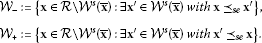2. (ii)

The unstable manifold ${\mathcal{W}}^{u}\left(\overline{\mathbf{x}}\right)$ is connected, and it is the graph of a continuous decreasing curve.

3. (iii)

For every $\mathbf{x}\in {\mathcal{W}}_{+}$, ${T}^{n}\left(\mathbf{x}\right)$ eventually enters the interior of the invariant set ${Q}_{4}\left(\overline{\mathbf{x}}\right)\cap \mathcal{R}$, and for every $\mathbf{x}\in {\mathcal{W}}_{-}$, ${T}^{n}\left(\mathbf{x}\right)$ eventually enters the interior of the invariant set ${Q}_{2}\left(\overline{\mathbf{x}}\right)\cap \mathcal{R}$.

4. (iv)

Let $\mathbf{m}\in {Q}_{2}\left(\overline{\mathbf{x}}\right)$ and $\mathbf{M}\in {Q}_{4}\left(\overline{\mathbf{x}}\right)$ be the endpoints of ${\mathcal{W}}^{u}\left(\overline{\mathbf{x}}\right)$, where $\mathbf{m}{⪯}_{se}\overline{\mathbf{x}}{⪯}_{se}\mathbf{M}$. For every $\mathbf{x}\in {\mathcal{W}}_{-}$ and every $\mathbf{z}\in \mathcal{R}$ such that $\mathbf{m}{⪯}_{se}z$, there exists $m\in \mathbb{N}$ such that ${T}^{m}\left(\mathbf{x}\right){⪯}_{se}z$, and for every $\mathbf{x}\in {\mathcal{W}}_{+}$ and every $\mathbf{z}\in \mathcal{R}$ such that $\mathbf{z}{⪯}_{se}\mathbf{M}$, there exists $m\in \mathbb{N}$ such that $\mathbf{M}{⪯}_{se}{T}^{m}\left(\mathbf{x}\right)$.

## 3 Linearized stability analysis

Lemma 1

1. (i)

If ${\beta }_{2}{\gamma }_{1}-{A}_{1}{A}_{2}\le 0$, then System (2) has a unique equilibrium point ${E}_{0}=\left(0,0\right)$.

2. (ii)

If ${\beta }_{2}{\gamma }_{1}-{A}_{1}{A}_{2}>0$, then System (2) has two equilibrium points ${E}_{0}$ and ${E}_{+}=\left(\overline{x},\overline{y}\right)$, $\overline{x}>0$, $\overline{y}>0$.

Proof The equilibrium point $E\left(\overline{x},\overline{y}\right)$ of System (2) satisfies the following system of equations:

$\overline{x}=\frac{{\gamma }_{1}\overline{y}}{{A}_{1}+\overline{x}},\phantom{\rule{2em}{0ex}}\overline{y}=\frac{{\beta }_{2}\overline{x}}{{A}_{2}+{B}_{2}\overline{x}+\overline{y}}.$
(4)

It is easy to see that ${E}_{0}=\left(0,0\right)$ is one equilibrium point for all values of the parameters, and ${E}_{+}=\left(\overline{x},\overline{y}\right)$ is a positive equilibrium point if ${\beta }_{2}{\gamma }_{1}-{A}_{1}{A}_{2}>0$. Indeed, substituting $\overline{y}$ from the first equation in (4) in the second equation in (4), we obtain that $\overline{x}$ satisfies the following equation:

$f\left(x\right)={x}^{3}+\left(2{A}_{1}+{B}_{2}{\gamma }_{1}\right){x}^{2}+\left({A}_{1}^{2}+{A}_{1}{B}_{2}{\gamma }_{1}+{A}_{2}{\gamma }_{1}\right)x+{\gamma }_{1}\left({A}_{1}{A}_{2}-{\beta }_{2}{\gamma }_{1}\right)=0.$
(5)

By using Descartes’ theorem, we have that equation (5) has one positive equilibrium if the condition

${\beta }_{2}{\gamma }_{1}-{A}_{1}{A}_{2}>0$
(6)

is satisfied, i.e., ${\beta }_{2}{\gamma }_{1}>{A}_{1}{A}_{2}$. □

Theorem 6

1. (i)

If ${\beta }_{2}{\gamma }_{1}<{A}_{1}{A}_{2}$, then ${E}_{0}$ is locally asymptotically stable.

2. (ii)

If ${\beta }_{2}{\gamma }_{1}={A}_{1}{A}_{2}$, then ${E}_{0}$ is non-hyperbolic.

3. (iii)

If ${\beta }_{2}{\gamma }_{1}>{A}_{1}{A}_{2}$, then ${E}_{0}$ is a repeller.

Proof The map T associated to System (2) is of the form (3). The Jacobian matrix of T at the equilibrium $E=\left(\overline{x},\overline{y}\right)$ is

${J}_{T}\left(\overline{x},\overline{y}\right)=\left(\begin{array}{cc}-\frac{{\gamma }_{1}\overline{y}}{{\left({A}_{1}+\overline{x}\right)}^{2}}& \frac{{\gamma }_{1}}{{A}_{1}+\overline{x}}\\ \frac{{\beta }_{2}\left({A}_{2}+\overline{y}\right)}{{\left({A}_{2}+{B}_{2}\overline{x}+\overline{y}\right)}^{2}}& -\frac{{\beta }_{2}\overline{x}}{{\left({A}_{2}+{B}_{2}\overline{x}+\overline{y}\right)}^{2}}\end{array}\right)$
(7)

and

${J}_{T}\left(0,0\right)=\left(\begin{array}{cc}0& \frac{{\gamma }_{1}}{{A}_{1}}\\ \frac{{\beta }_{2}}{{A}_{2}}& 0\end{array}\right).$

The corresponding characteristic equation has the following form:

${\lambda }^{2}-\frac{{\beta }_{2}{\gamma }_{1}}{{A}_{1}{A}_{2}}=0,$

from which ${\lambda }_{1,2}=±\sqrt{\frac{{\beta }_{2}{\gamma }_{1}}{{A}_{1}{A}_{2}}}$.

1. (i)

If ${\beta }_{2}{\gamma }_{1}<{A}_{1}{A}_{2}$, then $|{\lambda }_{1,2}|<1$, i.e., ${E}_{0}$ is locally asymptotically stable.

2. (ii)

If ${\beta }_{2}{\gamma }_{1}={A}_{1}{A}_{2}$, then $|{\lambda }_{1,2}|=1$, which implies that ${E}_{0}$ is non-hyperbolic.

3. (iii)

If ${\beta }_{2}{\gamma }_{1}>{A}_{1}{A}_{2}$, then $|{\lambda }_{1,2}|>1$, which implies that ${E}_{0}$ is a repeller.

□

Theorem 7

1. (1)

Assume that ${\beta }_{2}{\gamma }_{1}>{A}_{1}{A}_{2}$ and

${\beta }_{2}{\gamma }_{1}-{A}_{1}{A}_{2}>-{B}_{2}\left[{A}_{1}^{2}+{\gamma }_{1}\left({A}_{2}-{A}_{1}{B}_{2}\right)\right].$
(8)

Then the positive equilibrium ${E}_{+}$ is a saddle point.

1. (2)

Assume that

$0<{\beta }_{2}{\gamma }_{1}-{A}_{1}{A}_{2}=-{B}_{2}\left[{A}_{1}^{2}+{\gamma }_{1}\left({A}_{2}-{A}_{1}{B}_{2}\right)\right].$
(9)

Then the positive equilibrium ${E}_{+}$ is a non-hyperbolic point and

$\overline{x}=-{A}_{1}+\sqrt{{\gamma }_{1}\left({A}_{1}{B}_{2}-{A}_{2}\right)},\phantom{\rule{2em}{0ex}}\overline{y}=\frac{\left(-{A}_{1}+\sqrt{{\gamma }_{1}\left({A}_{1}{B}_{2}-{A}_{2}\right)}\right)\sqrt{{\gamma }_{1}\left({A}_{1}{B}_{2}-{A}_{2}\right)}}{{\gamma }_{1}}.$
1. (3)

Assume that

$0<{\beta }_{2}{\gamma }_{1}-{A}_{1}{A}_{2}<-{B}_{2}\left[{A}_{1}^{2}+{\gamma }_{1}\left({A}_{2}-{A}_{1}{B}_{2}\right)\right].$
(10)

Then the positive equilibrium ${E}_{+}$ is locally asymptotically stable.

Proof The Jacobian matrix of T at the equilibrium ${E}_{+}=\left(\overline{x},\overline{y}\right)$ is of the form (7) and the corresponding characteristic equation has the following form:

${\lambda }^{2}-p\lambda +q=0,$

where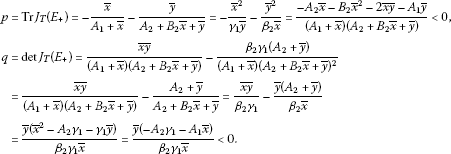Hence, for ${E}_{+}=\left(\overline{x},\overline{y}\right)$, we have $p<0$, $q<0$, so ${p}^{2}-4q>0$. Since

$\begin{array}{rcl}p-q-1& =& -\frac{{\overline{x}}^{2}}{{\gamma }_{1}\overline{y}}-\frac{{\overline{y}}^{2}}{{\beta }_{2}\overline{x}}-\frac{\overline{x}\overline{y}}{{\beta }_{2}{\gamma }_{1}}+\frac{\overline{y}\left({A}_{2}+\overline{y}\right)}{{\beta }_{2}\overline{x}}-1\stackrel{\left(\text{4}\right)}{=}-\frac{{\overline{x}}^{2}}{{\gamma }_{1}\overline{y}}-\frac{{\overline{y}}^{2}}{{\beta }_{2}\overline{x}}-\frac{\overline{x}\overline{y}}{{\beta }_{2}{\gamma }_{1}}+\left(1-\frac{{B}_{2}\overline{y}}{{\beta }_{2}}\right)-1\\ =& -\frac{{\overline{x}}^{2}}{{\gamma }_{1}\overline{y}}-\frac{{\overline{y}}^{2}}{{\beta }_{2}\overline{x}}-\frac{\overline{x}\overline{y}}{{\beta }_{2}{\gamma }_{1}}-\frac{{B}_{2}\overline{y}}{{\beta }_{2}}<0,\end{array}$

we obtain

$|p|\left\{\begin{array}{c}>|1+q|,\hfill \\ =|1+q|,\hfill \\ <|1+q|\hfill \end{array}⇔\phantom{\rule{1em}{0ex}}1+p+q\left\{\begin{array}{c}<0,\hfill \\ =0,\hfill \\ >0.\hfill \end{array}$

Similarly,

$\begin{array}{rcl}1+p+q& =& 1-\frac{\overline{x}}{{A}_{1}+\overline{x}}-\frac{\overline{y}}{{A}_{2}+{B}_{2}\overline{x}+\overline{y}}+\frac{\overline{x}\overline{y}}{\left({A}_{1}+\overline{x}\right)\left({A}_{2}+{B}_{2}\overline{x}+\overline{y}\right)}-\frac{{A}_{2}+\overline{y}}{{A}_{2}+{B}_{2}\overline{x}+\overline{y}}\\ =& -\frac{{A}_{2}\overline{x}+\overline{y}\left({A}_{1}+\overline{x}\right)-{A}_{1}{B}_{2}\overline{x}}{\left(\overline{x}+{A}_{1}\right)\left({A}_{2}+{B}_{2}\overline{x}+\overline{y}\right)}\\ \stackrel{\left(\text{4}\right)}{=}& -\frac{\overline{x}}{{\gamma }_{1}\left(\overline{x}+{A}_{1}\right)\left({A}_{2}+{B}_{2}\overline{x}+\overline{y}\right)}\varphi \left(\overline{x}\right),\end{array}$

where

Now, for the positive equilibrium, it holdsIf ${A}_{1}^{2}+{\gamma }_{1}\left({A}_{2}-{A}_{1}{B}_{2}\right)\ge 0$, then $\varphi \left(x\right)>0$ for all $x>0$, which implies that ${E}_{+}$ is a saddle point. If ${A}_{1}^{2}+{\gamma }_{1}\left({A}_{2}-{A}_{1}{B}_{2}\right)<0$, then $\varphi \left(x\right)=0$ for ${x}_{±}=-{A}_{1}±\sqrt{{\gamma }_{1}\left({A}_{1}{B}_{2}-{A}_{2}\right)}$ (${x}_{-}<0$, ${x}_{+}>0$).

Now we have three cases: ${x}_{+}<\overline{x}$, ${x}_{+}=\overline{x}$ or $\overline{x}<{x}_{+}$. Functions $f\left(x\right)$ and $\varphi \left(x\right)$ are increasing for $x>0$.

1. (1)

If ${x}_{+}<\overline{x}$, then $0=\varphi \left({x}_{+}\right)<\varphi \left(\overline{x}\right)$, i.e., $1+p+q<0$ and $f\left({x}_{+}\right). So,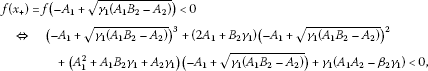from which it follows

${\gamma }_{1}{B}_{2}\left({A}_{1}{B}_{2}-{A}_{2}\right)<\left({\beta }_{2}{\gamma }_{1}-{A}_{1}{A}_{2}\right)+{A}_{1}^{2}{B}_{2},$

i.e.,

${\beta }_{2}{\gamma }_{1}>\left({A}_{1}-{\gamma }_{1}{B}_{2}\right)\left({A}_{2}-{A}_{1}{B}_{2}\right).$
(11)

Now we have

${\beta }_{2}{\gamma }_{1}-{A}_{1}{A}_{2}>-{B}_{2}\left[{A}_{1}^{2}+{\gamma }_{1}\left({A}_{2}-{A}_{1}{B}_{2}\right)\right],$

so we can see that the conditions (8) and (6) are sufficient for ${E}_{+}=\left(\overline{x},\overline{y}\right)$ to be a saddle point.

1. (2)

If ${x}_{+}=\overline{x}$, then $0=\varphi \left({x}_{+}\right)=\varphi \left(\overline{x}\right)$, hence $1+p+q=0$, i.e.,

$f\left({x}_{+}\right)=f\left(\overline{x}\right)=f\left(-{A}_{1}+\sqrt{{\gamma }_{1}\left({A}_{1}{B}_{2}-{A}_{2}\right)}\right)=0,$

from which

${\beta }_{2}{\gamma }_{1}=\left({A}_{1}-{\gamma }_{1}{B}_{2}\right)\left({A}_{2}-{A}_{1}{B}_{2}\right).$
(12)

If conditions (12) and (6) are satisfied, then

${\beta }_{2}{\gamma }_{2}-{A}_{1}{A}_{2}=-{B}_{2}\left[{A}_{1}^{2}+{\gamma }_{1}\left({A}_{2}-{A}_{1}{B}_{2}\right)\right]>0$

holds, i.e., ${E}_{+}=\left(\overline{x},\overline{y}\right)$ is a non-hyperbolic point of the form

$\overline{x}={x}_{+}=-{A}_{1}+\sqrt{{\gamma }_{1}\left({A}_{1}{B}_{2}-{A}_{2}\right)},\phantom{\rule{2em}{0ex}}\overline{y}=\frac{\left(-{A}_{1}+\sqrt{{\gamma }_{1}\left({A}_{1}{B}_{2}-{A}_{2}\right)}\right)\sqrt{{\gamma }_{1}\left({A}_{1}{B}_{2}-{A}_{2}\right)}}{{\gamma }_{1}}.$
1. (3)

If $\overline{x}<{x}_{+}$, then $\varphi \left(\overline{x}\right)<\varphi \left({x}_{+}\right)=0$ and

$0=f\left(\overline{x}\right)

from which

${\beta }_{2}{\gamma }_{1}<\left({A}_{1}-{\gamma }_{1}{B}_{2}\right)\left({A}_{2}-{A}_{1}{B}_{2}\right).$
(13)

Hence, if conditions (13) and (6) are satisfied, then

$0<{\beta }_{2}{\gamma }_{2}-{A}_{1}{A}_{2}<-{B}_{2}\left[{A}_{1}^{2}+{\gamma }_{1}\left({A}_{2}-{A}_{1}{B}_{2}\right)\right]$

holds, so ${E}_{+}$ is a locally asymptotically stable. □

## 4 Periodic character of solutions

In this section, we give the existence and local stability of period-two solutions.

Lemma 2 Assume that ${\beta }_{2}{\gamma }_{1}>{A}_{1}{A}_{2}$. Then System (2) has the following minimal period-two solutions:

${A}_{0}=\left(0,\frac{{\beta }_{2}{\gamma }_{1}-{A}_{1}{A}_{2}}{{\gamma }_{1}{B}_{2}}\right)\phantom{\rule{1em}{0ex}}\mathit{\text{and}}\phantom{\rule{1em}{0ex}}{B}_{0}=\left(\frac{{\beta }_{2}{\gamma }_{1}-{A}_{1}{A}_{2}}{{A}_{1}{B}_{2}},0\right).$
(14)

If

$0<{\beta }_{2}{\gamma }_{1}-{A}_{1}{A}_{2}=-{B}_{2}\left[{A}_{1}^{2}+{\gamma }_{1}\left({A}_{2}-{A}_{1}{B}_{2}\right)\right],$

then System (2) has an infinite number of minimal period-two solutions of the formfor $x\in \left[0,\frac{{\beta }_{2}{\gamma }_{1}-{A}_{1}{A}_{2}}{{A}_{1}{B}_{2}}\right]$, located along the line

$\mathcal{H}=\left\{\left(x,y\right):x{A}_{1}+{\gamma }_{1}y+{A}_{1}^{2}+{\gamma }_{1}\left({A}_{2}-{A}_{1}{B}_{2}\right)=0,x\in \left[0,\frac{{\beta }_{2}{\gamma }_{1}-{A}_{1}{A}_{2}}{{A}_{1}{B}_{2}}\right]\right\}.$
(15)

Proof The second iterate of T is (25). Equilibrium curves of the map ${T}^{2}\left(x,y\right)$ are

${C}_{1{T}^{2}}=\left\{\left(x,y\right)\in \left[0,\mathrm{\infty }{\right)}^{2}:x{\beta }_{2}{\gamma }_{1}\left(x+{A}_{1}\right)=x\left(y+{A}_{2}+x{B}_{2}\right)\left({A}_{1}^{2}+x{A}_{1}+y{\gamma }_{1}\right)\right\}$
(16)

and

$\begin{array}{rcl}{C}_{2{T}^{2}}& =& \left\{\left(x,y\right)\in \left[0,\mathrm{\infty }{\right)}^{2}:y{\beta }_{2}{\gamma }_{1}\left(y+{A}_{2}+x{B}_{2}\right)=y\left({A}_{1}{A}_{2}^{2}+{x}^{2}{\beta }_{2}+x{A}_{2}^{2}+{x}^{2}{A}_{2}{B}_{2}+xy{A}_{2}\\ +x{\beta }_{2}{A}_{1}+y{A}_{1}{A}_{2}+{y}^{2}{\gamma }_{1}{B}_{2}+y{\gamma }_{1}{A}_{2}{B}_{2}+x{A}_{1}{A}_{2}{B}_{2}+xy{\gamma }_{1}{B}_{2}^{2}\right)\right\}.\end{array}$
(17)

We get period-two solutions as the intersection point of equilibrium curves (16) and (17) in the first quadrant. If $x\ne 0$, $y=0$, then System (16), (17) is reduced to the equation

${\beta }_{2}{\gamma }_{1}\left(x+{A}_{1}\right)={A}_{1}\left({A}_{2}+x{B}_{2}\right)\left(x+{A}_{1}\right),$

and the positive solution of this equation is

If $x=0$, $y\ne 0$, then System (16), (17) is reduced to the equation

${\beta }_{2}{\gamma }_{1}\left(y+{A}_{2}\right)=\left(y+{A}_{2}\right)\left({A}_{1}{A}_{2}+y{\gamma }_{1}{B}_{2}\right),$

with the positive solution

On the other hand, if $x>0$, $y>0$, then we have

$\begin{array}{l}{\beta }_{2}{\gamma }_{1}\left(x+{A}_{1}\right)=\left(y+{A}_{2}+x{B}_{2}\right)\left({A}_{1}^{2}+x{A}_{1}+y{\gamma }_{1}\right)\\ {\beta }_{2}{\gamma }_{1}\left(y+{A}_{2}+x{B}_{2}\right)={A}_{1}{A}_{2}^{2}+{x}^{2}{\beta }_{2}+x{A}_{2}^{2}+{x}^{2}{A}_{2}{B}_{2}+xy{A}_{2}+x{\beta }_{2}{A}_{1}+y{A}_{1}{A}_{2}\\ \phantom{{\beta }_{2}{\gamma }_{1}\left(y+{A}_{2}+x{B}_{2}\right)=}+{y}^{2}{\gamma }_{1}{B}_{2}+y{\gamma }_{1}{A}_{2}{B}_{2}+x{A}_{1}{A}_{2}{B}_{2}+xy{\gamma }_{1}{B}_{2}^{2}\end{array}\right\},$

that is

$\left(x+{A}_{1}\right)\left({\beta }_{2}{\gamma }_{1}-{A}_{1}{A}_{2}\right)=\left(y+x{B}_{2}\right)\left({A}_{1}^{2}+x{A}_{1}+y{\gamma }_{1}\right)+y{\gamma }_{1}{A}_{2}$
(18)

and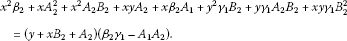(19)

Therefore, it must be $\left({\beta }_{2}{\gamma }_{1}-{A}_{1}{A}_{2}\right)>0$ in order to get any positive solution. By eliminating the term $\left({\beta }_{2}{\gamma }_{1}-{A}_{1}{A}_{2}\right)$ from (18) and using condition (9), we get

$\left(y+x{B}_{2}+{A}_{1}{B}_{2}\right)\left(y{\gamma }_{1}+x{A}_{1}+{A}_{1}^{2}+{\gamma }_{1}{A}_{2}-{\gamma }_{1}{A}_{1}{B}_{2}\right)=0,$

which implies

$y{\gamma }_{1}+x{A}_{1}+{A}_{1}^{2}+{\gamma }_{1}\left({A}_{2}-{A}_{1}{B}_{2}\right)=0,$

hence

$y=-\frac{1}{{\gamma }_{1}}\left(x{A}_{1}+{A}_{1}^{2}+{\gamma }_{1}\left({A}_{2}-{A}_{1}{B}_{2}\right)\right),\phantom{\rule{1em}{0ex}}{\gamma }_{1}\ne 0.$
(20)

Now, by eliminating y and the term $\left({A}_{1}{A}_{2}-{\beta }_{2}{\gamma }_{1}\right)$ from (19), we get the identity

$\left(x+{A}_{1}\right)\left(x+{A}_{1}-{\gamma }_{1}{B}_{2}\right)\frac{{\beta }_{2}{\gamma }_{1}-\left({A}_{2}-{A}_{1}{B}_{2}\right)\left({A}_{1}-{\gamma }_{1}{B}_{2}\right)}{{\gamma }_{1}}=0.$

If $x={\gamma }_{1}{B}_{2}-{A}_{1}$, we have

$y=-\frac{1}{{\gamma }_{1}}\left(x{A}_{1}+{A}_{1}^{2}+{\gamma }_{1}\left({A}_{2}-{A}_{1}{B}_{2}\right)\right)=-{A}_{2}<0,\phantom{\rule{1em}{0ex}}{\gamma }_{1}\ne 0.$

So, periodic solutions are located along line (15) with endpoints given by (14) using conditions (9). It is easy to see that ${A}_{x},{B}_{x}\in \mathcal{H}$ if ${\beta }_{2}{\gamma }_{1}-{A}_{1}{A}_{2}=-{B}_{2}\left[{A}_{1}^{2}+{\gamma }_{1}\left({A}_{2}-{A}_{1}{B}_{2}\right)\right]$. □

Let $\left(x,y\right)\in \mathcal{H}$, then the corresponding Jacobian matrix of the map ${T}^{2}$ has the following form:

${J}_{{T}^{2}}^{\mathcal{H}}\left(x,y\right)=\left(\begin{array}{cc}a& b\\ c& d\end{array}\right),$
(21)

where $a:={F}_{x}\left(x,y\right)$, $b:={F}_{y}\left(x,y\right)$, $c:={G}_{x}\left(x,y\right)$, $d:={G}_{y}\left(x,y\right)$.

Lemma 3 Assume that $0<{\beta }_{2}{\gamma }_{1}-{A}_{1}{A}_{2}=-{B}_{2}\left[{A}_{1}^{2}+{\gamma }_{1}\left({A}_{2}-{A}_{1}{B}_{2}\right)\right]$. Then the following statements are true.

1. (a)

The points ${A}_{x},{B}_{x}\in \mathcal{H}$ are non-hyperbolic fixed points for the map ${T}^{2}$, and both of them have eigenvalues ${\lambda }_{1}=1$ and ${\lambda }_{2}\in \left(0,1\right)$.

2. (b)

Eigenvectors corresponding to the eigenvalues ${\lambda }_{1}$ and ${\lambda }_{2}$ are not parallel to coordinate axes.

Proof (a) From (15) we have ${y}_{\mathcal{H}}^{\mathrm{\prime }}\left(x\right)=-\frac{{A}_{1}}{{\gamma }_{1}}<0$. Since

$\mathcal{H}=\left\{\left(x,y\right)\in \left[0,\mathrm{\infty }{\right)}^{2}:F\left(x,y\right)=x\right\}=\left\{\left(x,y\right)\in \left[0,\mathrm{\infty }{\right)}^{2}:G\left(x,y\right)=y\right\},$

by implicit differentiation of equations $F\left(x,y\right)=x$ and $G\left(x,y\right)=y$ at the point $\left(x,y\right)\in \mathcal{H}$, we obtain

${y}_{\mathcal{H}}^{\mathrm{\prime }}\left(x\right)=\frac{1-a}{b}=\frac{c}{1-d}=-\frac{{A}_{1}}{{\gamma }_{1}}<0.$
(22)

Since $a>0$, $b<0$, $c<0$ and $d>0$, from (22), we get

$0
(23)

The characteristic polynomial of the matrix (21) at the point $\left(x,y\right)\in \mathcal{H}$ is of the form

$P\left(\lambda \right)={\lambda }^{2}-\left(a+d\right)\lambda +\left(ad-bc\right).$

Now, using (22) we have $\left(1-a\right)\left(1-d\right)=bc$, and since

$P\left(1\right)=1-\left(a+d\right)+\left(ad-bc\right)=0,$

we get ${\lambda }_{1}=1$, and due to Vieta’s formulas and condition (23), it follows

$0<{\lambda }_{1}+{\lambda }_{2}=1+{\lambda }_{2}=a+d<2,$

i.e., $0<{\lambda }_{2}<1$.

(b) Eigenvectors corresponding to the eigenvalues ${\lambda }_{1}$ and ${\lambda }_{2}$ are ${\mathbf{v}}_{1}=\left(1-d,c\right)$ and ${\mathbf{v}}_{2}=\left(a-1,c\right)$. By condition (23) it is easy to see that these vectors are not parallel to the coordinate axes. □

Lemma 4 The periodic points ${A}_{0}$ and ${B}_{0}$ given by (14) are

1. (a)

locally asymptotically stable if ${\beta }_{2}{\gamma }_{1}-{A}_{1}{A}_{2}>-{B}_{2}\left[{A}_{1}^{2}+{\gamma }_{1}\left({A}_{2}-{A}_{1}{B}_{2}\right)\right]$ and ${\beta }_{2}{\gamma }_{1}>{A}_{1}{A}_{2}$,

2. (b)

non-hyperbolic if $0<{\beta }_{2}{\gamma }_{1}-{A}_{1}{A}_{2}=-{B}_{2}\left[{A}_{1}^{2}+{\gamma }_{1}\left({A}_{2}-{A}_{1}{B}_{2}\right)\right]$,

3. (c)

saddle points if $0<{\beta }_{2}{\gamma }_{1}-{A}_{1}{A}_{2}<-{B}_{2}\left[{A}_{1}^{2}+{\gamma }_{1}\left({A}_{2}-{A}_{1}{B}_{2}\right)\right]$.

Proof We have that

${J}_{{T}^{2}}\left(\frac{{\beta }_{2}{\gamma }_{1}-{A}_{1}{A}_{2}}{{A}_{1}{B}_{2}},0\right)=\left(\begin{array}{cc}\frac{{A}_{1}{A}_{2}}{{\beta }_{2}{\gamma }_{1}}& \frac{\left({\beta }_{2}{\gamma }_{1}-{A}_{1}{A}_{2}\right)\left({A}_{1}^{2}{A}_{2}-{A}_{1}^{3}{B}_{2}-{\beta }_{2}{\gamma }_{1}^{2}{B}_{2}-{\beta }_{2}{\gamma }_{1}{A}_{1}\right)}{{\beta }_{2}{\gamma }_{1}{A}_{1}{B}_{2}\left({A}_{1}^{2}{B}_{2}+{\beta }_{2}{\gamma }_{1}-{A}_{1}{A}_{2}\right)}\\ 0& \frac{{\beta }_{2}{\gamma }_{1}^{2}{A}_{1}{B}_{2}^{2}}{\left({A}_{1}^{2}{B}_{2}+{\beta }_{2}{\gamma }_{1}-{A}_{1}{A}_{2}\right)\left({\beta }_{2}{\gamma }_{1}-{A}_{1}{A}_{2}+{\gamma }_{1}{A}_{2}{B}_{2}\right)}\end{array}\right)$

and characteristic eigenvalues are

${\lambda }_{1}=\frac{{A}_{1}{A}_{2}}{{\beta }_{2}{\gamma }_{1}}<1\phantom{\rule{1em}{0ex}}\text{and}\phantom{\rule{1em}{0ex}}{\lambda }_{2}=\frac{{\beta }_{2}{\gamma }_{1}^{2}{A}_{1}{B}_{2}^{2}}{\left({\beta }_{2}{\gamma }_{1}-{A}_{1}{A}_{2}+{\gamma }_{1}{A}_{2}{B}_{2}\right)\left({B}_{2}{A}_{1}^{2}-{A}_{2}{A}_{1}+{\beta }_{2}{\gamma }_{1}\right)}.$

Now,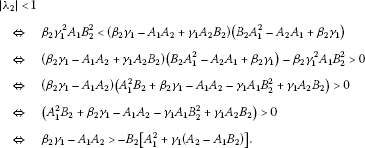Therefore,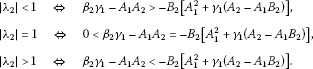On the other hand, we have

${J}_{{T}^{2}}\left(0,\frac{{\beta }_{2}{\gamma }_{1}-{A}_{1}{A}_{2}}{{\gamma }_{1}{B}_{2}}\right)=\left(\begin{array}{cc}\frac{{\beta }_{2}{\gamma }_{1}^{2}{A}_{1}{B}_{2}^{2}}{\left({A}_{1}^{2}{B}_{2}+{\beta }_{2}{\gamma }_{1}-{A}_{1}{A}_{2}\right)\left({\beta }_{2}{\gamma }_{1}-{A}_{1}{A}_{2}+{\gamma }_{1}{A}_{2}{B}_{2}\right)}& 0\\ \frac{\left({\beta }_{2}{\gamma }_{1}-{A}_{1}{A}_{2}\right)\left({A}_{1}{A}_{2}^{2}-{\gamma }_{1}{A}_{2}^{2}{B}_{2}-{\beta }_{2}{\gamma }_{1}{A}_{2}-{\beta }_{2}{\gamma }_{1}{A}_{1}{B}_{2}\right)}{{\beta }_{2}{\gamma }_{1}^{2}{B}_{2}\left({\beta }_{2}{\gamma }_{1}-{A}_{1}{A}_{2}+{\gamma }_{1}{A}_{2}{B}_{2}\right)}& \frac{{A}_{1}{A}_{2}}{{\beta }_{2}{\gamma }_{1}}\end{array}\right)$

and the corresponding eigenvalues are

${\lambda }_{1}=\frac{{A}_{1}{A}_{2}}{{\beta }_{2}{\gamma }_{1}}<1\phantom{\rule{1em}{0ex}}\text{and}\phantom{\rule{1em}{0ex}}{\lambda }_{2}=\frac{{\beta }_{2}{\gamma }_{1}^{2}{A}_{1}{B}_{2}^{2}}{\left({\beta }_{2}{\gamma }_{1}-{A}_{1}{A}_{2}+{\gamma }_{1}{A}_{2}{B}_{2}\right)\left({B}_{2}{A}_{1}^{2}-{A}_{2}{A}_{1}+{\beta }_{2}{\gamma }_{1}\right)},$

so it comes to the same conclusion! □

## 5 Global results

In this section, we present the results on the global dynamics of System (2).

Lemma 5 Every solution of System (2) satisfies

1. 1.

${x}_{n}\le \frac{{\gamma }_{1}}{{A}_{1}}\cdot \frac{{\beta }_{2}}{{B}_{2}}$, ${y}_{n}\le \frac{{\beta }_{2}}{{B}_{2}}$, $n=2,3,\dots$.

2. 2.

If ${\beta }_{2}{\gamma }_{1}<{A}_{1}{A}_{2}$, then ${lim}_{n\to \mathrm{\infty }}{x}_{n}=0$, ${lim}_{n\to \mathrm{\infty }}{y}_{n}=0$.

The map T satisfies:

1. 3.

$T\left(\mathcal{B}\right)\subseteq \mathcal{B}$, where $\mathcal{B}=\left[0,\frac{{\gamma }_{1}}{{A}_{1}}\cdot \frac{{\beta }_{2}}{{B}_{2}}\right]×\left[0,\frac{{\beta }_{2}}{{B}_{2}}\right]$, that is, $\mathcal{B}$ is an invariant box.

2. 4.

$T\left(\mathcal{B}\right)$ is an attracting box, that is $T{\left(\left[0,\mathrm{\infty }\right)}^{2}\right)\subseteq \mathcal{B}$.

Proof From System (2), we have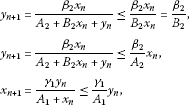for $n=0,1,2,\dots$ , and

${x}_{n+1}\le \frac{{\gamma }_{1}}{{A}_{1}}{y}_{n}\le \frac{{\gamma }_{1}}{{A}_{1}}\cdot \frac{{\beta }_{2}}{{B}_{2}}$

for $n=1,2,\dots$ . Furthermore, we get

${x}_{n}\le \frac{{\gamma }_{1}}{{A}_{1}}{y}_{n-1}\le \frac{{\gamma }_{1}{\beta }_{2}}{{A}_{1}{A}_{2}}{x}_{n-2},$

i.e.,

${x}_{2n}\le {\left(\frac{{\gamma }_{1}{\beta }_{2}}{{A}_{1}{A}_{2}}\right)}^{n}{x}_{0},\phantom{\rule{2em}{0ex}}{x}_{2n+1}\le {\left(\frac{{\gamma }_{1}{\beta }_{2}}{{A}_{1}{A}_{2}}\right)}^{n}{x}_{1},$

so it follows that ${lim}_{n\to \mathrm{\infty }}{x}_{n}=0$, ${lim}_{n\to \mathrm{\infty }}{y}_{n}=0$ if ${\beta }_{2}{\gamma }_{1}<{A}_{1}{A}_{2}$.

Proof of 3. and 4. is an immediate checking. □

Lemma 6 The map ${T}^{2}$ is injective and $det{J}_{{T}^{2}}\left(x,y\right)>0$, for all $x\ge 0$ and $y\ge 0$.

Proof (i) Here we prove that map T is injective, which implies that ${T}^{2}$ is injective. Indeed, $T\left(\begin{array}{c}{x}_{1}\\ {y}_{1}\end{array}\right)=T\left(\begin{array}{c}{x}_{2}\\ {y}_{2}\end{array}\right)$ implies that

${A}_{1}\left({y}_{1}-{y}_{2}\right)={x}_{1}{y}_{2}-{x}_{2}{y}_{1},\phantom{\rule{2em}{0ex}}{A}_{2}\left({x}_{1}-{x}_{2}\right)={x}_{2}{y}_{1}-{x}_{1}{y}_{2}.$
(24)

By solving System (24) with respect to ${x}_{1}$, ${x}_{2}$ or ${y}_{1}$, ${y}_{2}$, we obtain that $\left({x}_{1},{y}_{1}\right)=\left({x}_{2},{y}_{2}\right)$.

1. (ii)

The map ${T}^{2}\left(x,y\right)=\left(\begin{array}{c}F\left(x,y\right)\\ G\left(x,y\right)\end{array}\right)$ is of the form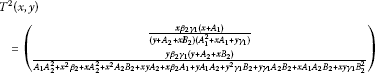(25)

and

${J}_{{T}^{2}}\left(x,y\right)=\left(\begin{array}{cc}{F}_{x}& {F}_{y}\\ {G}_{x}& {G}_{y}\end{array}\right),$

where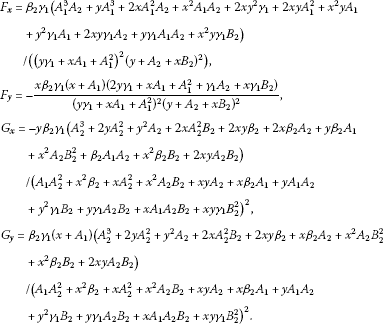Now, we obtain

$det{J}_{{T}^{2}}\left(x,y\right)={F}_{x}{G}_{y}-{F}_{y}{G}_{x}=UV,$

where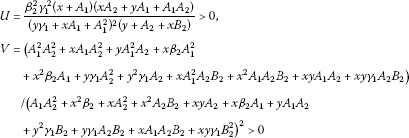and the Jacobian matrix of ${T}^{2}\left(x,y\right)$ is invertible for all $x\ge 0$ and $y\ge 0$. □

Corollary 1 The competitive map ${T}^{2}$ satisfies the condition (O+). Consequently, the sequences $\left\{{x}_{2n}\right\}$, $\left\{{x}_{2n+1}\right\}$, $\left\{{y}_{2n}\right\}$, $\left\{{y}_{2n+1}\right\}$ of every solution of System (2) are eventually monotone.

Proof It immediately follows from Lemma 6, Theorem 2 and 3. □

Lemma 7 Assume ${\beta }_{2}{\gamma }_{1}-{A}_{1}{A}_{2}>0$. System (2) has period-two solutions (14) and

1. (a)

If $\left({x}_{0},{y}_{0}\right)=\left(x,0\right)$, $x>0$, then

$\underset{n\to \mathrm{\infty }}{lim}{T}^{2n}\left(x,0\right)=\left(\frac{{\beta }_{2}{\gamma }_{1}-{A}_{1}{A}_{2}}{{A}_{1}{B}_{2}},0\right)={B}_{0}$

and

$\underset{n\to \mathrm{\infty }}{lim}{T}^{2n+1}\left(x,0\right)=\left(0,\frac{{\beta }_{2}{\gamma }_{1}-{A}_{1}{A}_{2}}{{\gamma }_{1}{B}_{2}}\right)={A}_{0}.$
1. (b)

If $\left({x}_{0},{y}_{0}\right)=\left(0,y\right)$, $y>0$, then

$\underset{n\to \mathrm{\infty }}{lim}{T}^{2n}\left(0,y\right)=\left(0,\frac{{\beta }_{2}{\gamma }_{1}-{A}_{1}{A}_{2}}{{\gamma }_{1}{B}_{2}}\right)={A}_{0}$

and

$\underset{n\to \mathrm{\infty }}{lim}{T}^{2n+1}\left(x,0\right)=\left(\frac{{\beta }_{2}{\gamma }_{1}-{A}_{1}{A}_{2}}{{A}_{1}{B}_{2}},0\right)={B}_{0}.$

Proof (a) For all $x>0$, $x\ne \frac{{\beta }_{2}{\gamma }_{1}-{A}_{1}{A}_{2}}{{A}_{1}{B}_{2}}$, we have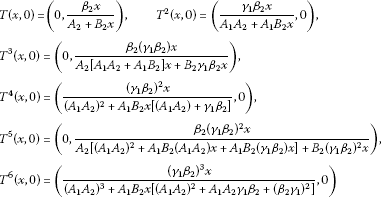and by induction,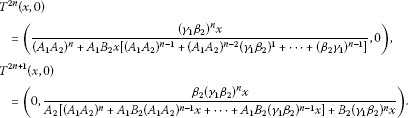Now, we have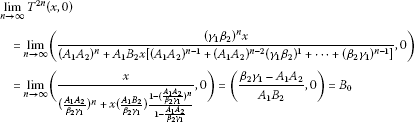and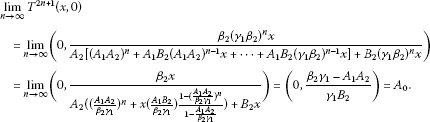□

Lemma 8 The map ${T}^{2}$ associated to System (2) satisfies the following:

${T}^{2}\left(x,y\right)=\left(\overline{x},\overline{y}\right)\phantom{\rule{1em}{0ex}}\mathit{\text{only for}}\phantom{\rule{0.25em}{0ex}}\left(x,y\right)=\left(\overline{x},\overline{y}\right).$

Proof Since ${T}^{2}$ is injective, then ${T}^{2}\left(x,y\right)=\left(\overline{x},\overline{y}\right)={T}^{2}\left(\overline{x},\overline{y}\right)⇒\left(x,y\right)=\left(\overline{x},\overline{y}\right)$. □

Proof of Theorem 1 Case 1 ${\beta }_{2}{\gamma }_{1}\le {A}_{1}{A}_{2}$

Equilibrium ${E}_{0}$ is unique (see Lemma 1), and by Lemma 5, every solution of System (2) belongs to

$B=\left[\begin{array}{c}0,\frac{{\beta }_{2}{\gamma }_{1}}{{A}_{1}{B}_{2}}\end{array}\right]×\left[\begin{array}{c}0,\frac{{\beta }_{2}}{{B}_{2}}\end{array}\right],$

which is an invariant box. In view of Corollary 1 and Theorem 2, every solution converges to minimal period-two solutions or ${E}_{0}$. System (2) has no minimal period-two solutions (Lemma 2). So, every solution of System (2) converges to ${E}_{0}$.

Case 2 ${\beta }_{2}{\gamma }_{1}-{A}_{1}{A}_{2}>-{B}_{2}\left[{A}_{1}^{2}+{\gamma }_{1}\left({A}_{2}-{A}_{1}{B}_{2}\right)\right]$ and ${\beta }_{2}{\gamma }_{1}-{A}_{1}{A}_{2}>0$

By Lemmas 1, 2, 4 and Theorems 6 and 7, there exist two equilibrium points: ${E}_{0}$ which is a repeller and ${E}_{+}$ which is a saddle point, and minimal period-two solutions ${A}_{0}$ and ${B}_{0}$ which are locally asymptotically stable. Clearly ${T}^{2}$ is strongly competitive and it is easy to check that the points ${A}_{0}$ and ${B}_{0}$ are locally asymptotically stable for ${T}^{2}$ as well. System (2) can be decomposed into the system of the even-indexed and odd-indexed terms as follows:

$\left\{\begin{array}{c}{x}_{2n+1}=\frac{{\gamma }_{1}{y}_{2n}}{{A}_{1}+{x}_{2n}},\hfill \\ {x}_{2n}=\frac{{\gamma }_{1}{y}_{2n-1}}{{A}_{1}+{x}_{2n-1}},\hfill \\ {y}_{2n+1}=\frac{{\beta }_{2}{x}_{2n}}{{A}_{2}+{B}_{2}{x}_{2n}+{y}_{2n}},\hfill \\ {y}_{2n}=\frac{{\beta }_{2}{x}_{2n-1}}{{A}_{2}+{B}_{2}{x}_{2n-1}+{y}_{2n-1}},\phantom{\rule{1em}{0ex}}n=1,2,\dots .\hfill \end{array}$

The existence of the set $\mathcal{C}$ with the stated properties follows from Lemmas 6, 2, 7, 8, Corollary 1, Theorems 4 and 5.

Case 3 $0<{\beta }_{2}{\gamma }_{1}-{A}_{1}{A}_{2}=-{B}_{2}\left[{A}_{1}^{2}+{\gamma }_{1}\left({A}_{2}-{A}_{1}{B}_{2}\right)\right]$

Cases (i) and (ii) from (c) in Theorem 1 are the consequence of Lemmas 1, 2, 4 and Theorems 6 and 7.

Since ${T}^{2}$ is strongly competitive and points ${A}_{x}$ and ${B}_{x}$, for all $x\in \left[0,\frac{{\beta }_{2}{\gamma }_{1}-{A}_{1}{A}_{2}}{{A}_{1}{B}_{2}}\right)$, are non-hyperbolic points of the map ${T}^{2}$, by Lemmas 1, 6, 2, 3, 7, Corollary 1, Theorems 2, 5, 6 and 7, it follows that all conditions of Theorem 4 are satisfied for the map ${T}^{2}$ with $\mathcal{R}=\left[0,\mathrm{\infty }\right)×\left[0,\mathrm{\infty }\right)$. By Lemma 7, it is clear that

${\mathcal{C}}_{{A}_{0}}=\left\{\left(x,y\right):x=0,y>0\right\}\phantom{\rule{1em}{0ex}}\text{and}\phantom{\rule{1em}{0ex}}{\mathcal{C}}_{{B}_{0}}=\left\{\left(x,y\right):x>0,y=0\right\}.$

Case 4 $0<{\beta }_{2}{\gamma }_{1}-{A}_{1}{A}_{2}<-{B}_{2}\left[{A}_{1}^{2}+{\gamma }_{1}\left({A}_{2}-{A}_{1}{B}_{2}\right)\right]$

Lemma 2 implies that System (2) has minimal period-two solutions (14). Furthermore, Corollary 1 and Theorem 2 imply that all solutions of System (2) converge to an equilibrium or minimal period-two solutions, and since, by Theorem 6, ${E}_{0}$ is a repeller, all solutions converge to ${E}_{+}$ (which is, in view of Theorem 7, locally asymptotically stable) or minimal period-two solutions (14). The points ${A}_{0}$ and ${B}_{0}$ are saddle points of the strongly competitive map ${T}^{2}$; and by Lemma 7, the stable manifold of ${A}_{0}$ (under ${T}^{2}$) is

$\mathcal{B}\left({A}_{0}\right)=\left\{\left(x,y\right):x=0,y>0\right\}$

and the stable manifold of ${B}_{0}$ (under ${T}^{2}$) is

$\mathcal{B}\left({B}_{0}\right)=\left\{\left(x,y\right):x>0,y=0\right\}$

and each of these stable manifolds is unique. This implies that the basin of attraction of the equilibrium point ${E}_{+}$ is the set

$\mathcal{B}\left({E}_{+}\right)=\left\{\left(x,y\right):x>0,y>0\right\},$

and Lemma 7 completes the conclusion (d) of Theorem 1. □

## References

1. Camouzis E, Kulenović MRS, Ladas G, Merino O: Rational systems in the plane - open problems and conjectures. J. Differ. Equ. Appl. 2009, 15: 303–323. 10.1080/10236190802125264

2. Clark D, Kulenović MRS: On a coupled system of rational difference equations. Comput. Math. Appl. 2002, 43: 849–867. 10.1016/S0898-1221(01)00326-1

3. Clark CA, Kulenović MRS, Selgrade JF: On a system of rational difference equations. J. Differ. Equ. Appl. 2005, 11: 565–580. 10.1080/10236190412331334464

4. Hirsch M, Smith H: Monotone Dynamical Systems. Ordinary Differential Equations 2. In Handbook of Differential Equations. Elsevier, Amsterdam; 2005:239–357.

5. Kulenović MRS, Ladas G: Dynamics of Second Order Rational Difference Equations with Open Problems and Conjectures. Chapman and Hall/CRC, Boca Raton; 2001.

6. Kulenović MRS, Merino O: Discrete Dynamical Systems and Difference Equations with Mathematica. Chapman and Hall/CRC, Boca Raton; 2002.

7. Kulenović MRS, Nurkanović M: Asymptotic behavior of a system of linear fractional difference equations. J. Inequal. Appl. 2005, 2005: 127–144.

8. Kulenović MRS, Nurkanović M: Asymptotic behavior of a competitive system of linear fractional difference equations. Adv. Differ. Equ. 2006, 2006: 1–13.

9. Brett A, Garić-Demirović M, Kulenović MRS, Nurkanović M: Global behavior of two competitive rational systems of difference equations in the plane. Commun. Appl. Nonlinear Anal. 2009, 16: 1–18.

10. Garic-Demirović M, Kulenović MRS, Nurkanović M: Global behavior of four competitive rational systems of difference equations in the plane. Discrete Dyn. Nat. Soc. 2009., 2009: Article ID 153058

11. Smith HL: Planar competitive and cooperative difference equations. J. Differ. Equ. Appl. 1998, 3: 335–357. 10.1080/10236199708808108

12. Smith HL: The discrete dynamics of monotonically decomposable maps. J. Math. Biol. 2006, 53: 747–758. 10.1007/s00285-006-0004-3

13. Kalabušić S, Kulenović MRS: Dynamics of certain anti-competitive systems of rational difference equations in the plane. J. Differ. Equ. Appl. 2011, 17: 1599–1615. 10.1080/10236191003730506

14. Garic-Demirović M, Nurkanović M: Dynamics of an anti-competitive two dimensional rational system of difference equations. Sarajevo J. Math. 2011, 7(19):39–56.

15. Kalabušić, S, Kulenović, MRS, Pilav, E: Global dynamics of anti-competitive systems in the plane (submitted)

16. Kulenović MRS, Merino O: Competitive-exclusion versus competitive-coexistence for systems in the plane. Discrete Contin. Dyn. Syst., Ser. B 2006, 6: 1141–1156.

17. Kulenović MRS, Merino O: Global bifurcation for discrete competitive systems in the plane. Discrete Contin. Dyn. Syst., Ser. B 2009, 12: 133–149.

18. Robinson C: Stability, Symbolic Dynamics, and Chaos. CRC Press, Boca Raton; 1995.

19. Kulenović MRS, Merino O: Invariant manifolds for competitive discrete systems in the plane. Int. J. Bifurc. Chaos 2010, 20: 2471–2486. 10.1142/S0218127410027118

20. Clark D, Kulenović MRS, Selgrade JF: Global asymptotic behavior of a two dimensional difference equation modelling competition. Nonlinear Anal. TMA 2003, 52: 1765–1776. 10.1016/S0362-546X(02)00294-8

21. Kulenović MRS, Merino O: A global attractivity result for maps with invariant boxes. Discrete Contin. Dyn. Syst., Ser. B 2006, 6: 97–110.

## Acknowledgements

The authors are very grateful to Professor M.R.S. Kulenović for his valuable suggestions. They thank also the referees for their useful comments.

## Author information

Authors

### Corresponding author

Correspondence to Zehra Nurkanović.

### Competing interests

The authors declare that they have no competing interests.

### Authors’ contributions

Both authors contributed to each part of this study equally and read and approved the final version of the manuscript.

## Authors’ original submitted files for images

Below are the links to the authors’ original submitted files for images.

## Rights and permissions

Reprints and Permissions

Moranjkić, S., Nurkanović, Z. Basins of attraction of certain rational anti-competitive system of difference equations in the plane. Adv Differ Equ 2012, 153 (2012). https://doi.org/10.1186/1687-1847-2012-153

• Accepted:

• Published:

• DOI: https://doi.org/10.1186/1687-1847-2012-153

### Keywords

• difference equations
• anti-competitive
• map
• stability
• stable manifold